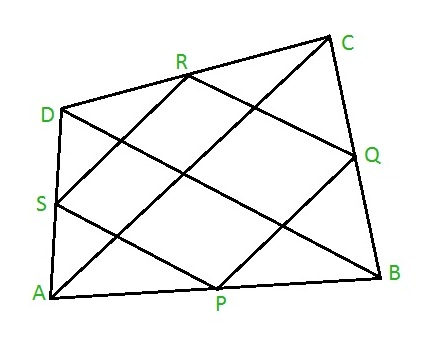# Perimeter and Area of Varignon’s Parallelogram

• Last Updated : 11 Jan, 2022

Given a and b are the lengths of the diagonals AC and BD of a quadrilateral ABCD with the area of quadrilateral as s. The task is to find the perimeter and area of the Varignon’s parallelogram PQRS.

Note: When we join the mid-points of the sides of any quadrilateral, the new quadrilateral formed inside will always be a parallelogram and this parallelogram is known as the Varignon’s parallelogram named upon the French Mathematician Pierre Varignon. Thus, PQRS will be a parallelogram since it is formed by joining the mid-points of quadrilateral ABCD as shown below:Example:

Input: a = 7, b = 8, s = 10
Output: Perimeter = 15, Area = 5

Approach: The perimeter of Varignon’s parallelogram PQRS is equal to the sum of the length of the diagonals of quadrilateral ABCD.
Hence, Perimeter = a + b, where a and b are lengths of diagonals AC and BD.
Also, the area of the Varignon’s parallelogram is always half the area of quadrilateral ABCD.
Hence, Area = s / 2, where s is the area of quadrilateral ABCD.

Below is the implementation of the above approach:

## C

 `// C program to find the perimeter and area``#include ` `// Function to find the perimeter``float` `per( ``float` `a, ``float` `b )``{``    ``return` `( a + b );``}` `// Function to find the area``float` `area( ``float` `s )``{``    ``return` `( s/2 );``}` `// Driver code``int` `main()``{``    ``float` `a = 7, b = 8, s = 10;``    ``printf``(``"%f\n"``,``           ``per( a, b ));``    ``printf``(``"%f"``,``           ``area( s ));``    ``return` `0;``}`

## Java

 `// Java code to find the perimeter and area``import` `java.lang.*;` `class` `GFG {` `    ``// Function to find the perimeter``    ``public` `static` `double` `per(``double` `a, ``double` `b)``    ``{``        ``return` `(a + b);``    ``}``    ``// Function to find the area``    ``public` `static` `double` `area(``double` `s)``    ``{``        ``return` `(s / ``2``);``    ``}` `    ``// Driver code``    ``public` `static` `void` `main(String[] args)``    ``{``        ``double` `a = ``7``, b = ``8``, s = ``10``;``        ``System.out.println(per(a, b));``        ``System.out.println(area(s));``    ``}``}`

## Python3

 `# Python3 code to find the perimeter and area``    ` `# Function to find the perimeter``def` `per( a, b ):``    ``return` `( a ``+` `b )``# Function to find the area``def` `area( s ):``    ``return` `( s ``/` `2` `)``    ` `# Driver code``a ``=` `7``b ``=` `8``s ``=` `10``print``( per( a, b ))``print``( area( s ))`

## C#

 `// C# code to find the perimeter and area``using` `System;` `class` `GFG {` `    ``// Function to find the perimeter``    ``public` `static` `double` `per(``double` `a, ``double` `b)``    ``{``        ``return` `(a + b);``    ``}``    ``// Function to find the area``    ``public` `static` `double` `area(``double` `s)``    ``{``        ``return` `(s / 2);``    ``}` `    ``// Driver code``    ``public` `static` `void` `Main()``    ``{``        ``double` `a = 7.0, b = 8.0, s = 10.0;``        ``Console.WriteLine(per(a, b));``        ``Console.Write(area(s));``    ``}``}`

## PHP

 ``

## Javascript

 ``
Output:
```15
5```

My Personal Notes arrow_drop_up